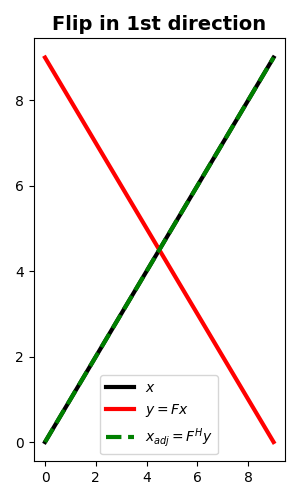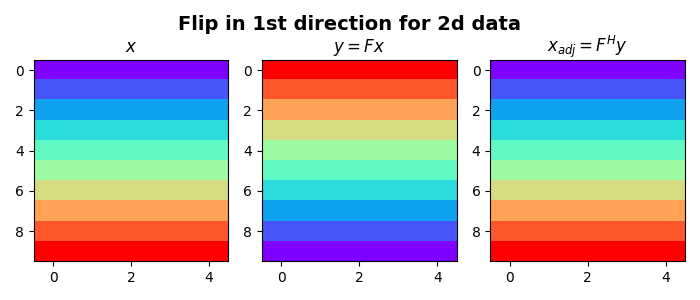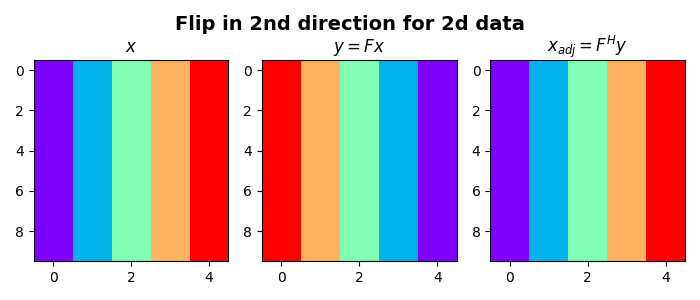# Flip along an axis#

This example shows how to use the `pylops.Flip` operator to simply flip an input signal along an axis.

```import matplotlib.pyplot as plt
import numpy as np

import pylops

plt.close("all")
```

Let’s start with a 1D example. Define an input signal composed of `nt` samples

```nt = 10
x = np.arange(nt)
```

We can now create our flip operator and apply it to the input signal. We can also apply the adjoint to the flipped signal and we can see how for this operator the adjoint is effectively equivalent to the inverse.

```Fop = pylops.Flip(nt)
y = Fop * x

plt.figure(figsize=(3, 5))
plt.plot(x, "k", lw=3, label=r"\$x\$")
plt.plot(y, "r", lw=3, label=r"\$y=Fx\$")
plt.title("Flip in 1st direction", fontsize=14, fontweight="bold")
plt.legend()
plt.tight_layout()
```Let’s now repeat the same exercise on a two dimensional signal. We will first flip the model along the first axis and then along the second axis

```nt, nx = 10, 5
x = np.outer(np.arange(nt), np.ones(nx))
Fop = pylops.Flip((nt, nx), axis=0)
y = Fop * x

fig, axs = plt.subplots(1, 3, figsize=(7, 3))
fig.suptitle(
"Flip in 1st direction for 2d data", fontsize=14, fontweight="bold", y=0.95
)
axs.imshow(x, cmap="rainbow")
axs.set_title(r"\$x\$")
axs.axis("tight")
axs.imshow(y, cmap="rainbow")
axs.set_title(r"\$y = F x\$")
axs.axis("tight")
axs.axis("tight")
plt.tight_layout()

x = np.outer(np.ones(nt), np.arange(nx))
Fop = pylops.Flip(dims=(nt, nx), axis=1)
y = Fop * x

# sphinx_gallery_thumbnail_number = 3
fig, axs = plt.subplots(1, 3, figsize=(7, 3))
fig.suptitle(
"Flip in 2nd direction for 2d data", fontsize=14, fontweight="bold", y=0.95
)
axs.imshow(x, cmap="rainbow")
axs.set_title(r"\$x\$")
axs.axis("tight")
axs.imshow(y, cmap="rainbow")
axs.set_title(r"\$y = F x\$")
axs.axis("tight")
••